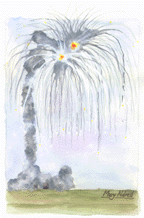# The Ariane 5 explosion as seen by a software engineer### Lack of attention to the strict preconditions below, especially the last term in each, was the direct cause of the destruction of the Ariane 5 and its payload -- a loss of approximately DM 1200 million.

strict precondition 1:
{Set."x"=FLPT and Set."y"=INT16 and -32768 <= x <= +32767}
strict precondition 2:
{Set."x"=FLPT and Set."y"=INT16 and int(x) in INT16}
strict precondition 3:
{Set."x"=FLPT and Set."y"=INT16 and int(x) in Set."y"}
program code (convert floating point x to fixed point integer y):
y := int(x)
postcondition:
{Set."x"=FLPT and Set."y"=INT16 and y=int(x)}
The error which ultimately led to the destruction of the Ariane 5 launcher about 40 seconds after lift off on its maiden flight was clearly identified in the report of the investigating committee : a program segment for converting a floating point number to a signed 16 bit integer was executed with an input data value outside the range representable by a signed 16 bit integer. This run time error (out of range, overflow), which arose in both the active and the backup computers at about the same time, was detected and both computers shut themselves down. This resulted in the total loss of attitude control. The Ariane 5 turned uncontrollably and aerodynamic forces broke the vehicle apart. This breakup was detected by an on-board monitor which ignited the explosive charges to destroy the vehicle in the air. Ironically, the result of this format conversion was no longer needed after lift off.

It would seem likely that the programmer of the subprogram for converting a floating point number to a signed 16 bit number realized that the value of the floating point number to be converted must lie within a restricted range, namely the range of values which (after truncating or rounding to an integer) can be represented as a signed 16 bit number. Such restrictions on input values (preconditions for subprograms) were, however, neither systematically derived, documented nor followed back to determine corresponding restrictions on other values computed earlier .

An analysis of this anomaly in Ariane 5's software represents a rather simple, almost trivial application of correctness proof techniques. The conversion of a floating point number to a signed 16 bit integer can be represented as the single assignment statement y:=int(x), where the variable x is (previously) declared as a floating point number and y is declared as a signed 16 bit integer. That is, the values of x are taken from the set FLTP of floating point numbers and the values of y are taken from the set INT16 of signed 16 bit integers. The function int maps a number to an integer in a suitable way. After execution of the conversion routine (the assignment statement above) the variables x and y will still be declared and the value of y should be the value of int(x), i.e. the postcondition of the conversion routine is {Set."x"=FLPT and Set."y"=INT16 and y=int(x)}.

A precondition can be derived by applying a standard proof rule for an assignment statement. An ordinary precondition (one which does not necessarily guarantee the successful execution of the assignment statement) can be found by applying the well known proof rule for an assignment statement. We require, however, a strict precondition, i.e. one which does guarantee successful execution of the assignment statement, so must use the proof rule for a strict precondition [2, 3]:

{Pzexpr and expr in Set."z"} z := expr {P} strictly

Applying this proof rule to the postcondition of the conversion routine gives the third precondition shown above, which is equivalent to the second precondition shown there. Assuming that the set INT16 is the set of consecutive integers from -32768 to +32767 inclusive and assuming only that the function int maps a non-integer argument to either of the neighboring integers and an integer argument to itself, the second precondition can be strengthened minimally to the first precondition shown above. Just before the end of the flight of the Ariane 5 the conversion routine was, clearly, executed with a value of x which violated this precondition, leading ultimately to the destruction of the vehicle and the failure of the mission.

The loss was estimated at DM 1200 million .

The pre-engineering days of other fields exhibited similar mishaps. For example, in 1628 the ship Wasa sank shortly after launching. Also the cause was similar: Her designers did not know how to calculate her stability. They had a good excuse, though: The necessary theory -- Newton's laws of mechanics -- had not yet been discovered. The developers of the software for the Ariane 5 do not have quite so convenient an excuse.

References:

 ARIANE 5 Flight 501 Failure, Report by the Inquiry Board, http://sspg1.bnsc.rl.ac.uk/Share/ISTP/ariane5r.htm (40 KB), http://ravel.esrin.esa.it/docs/esa-x-1819eng.pdf (2 MB), Paris, 1996 July 19.

 Baber, Robert L.; Praktische Anwendbarkeit mathematisch rigoroser Methoden zum Sicherstellen der Programmkorrektheit, Reihe Programmierung komplexer Systeme / PKS, see http://Baber.servehttp.com/Books/Books.html, Walter de Gruyter, Berlin, 1995.

 Baber, Robert L.; Lecture notes, exercises, etc. for the course "Introduction to Mathematically Rigorous Software Design", http://www.cas.mcmaster.ca/~baber/Courses/46L03.

 "Nachlässigkeit war der Grund für Desaster beim Erstflug der Ariane 5", VDI Nachrichten, 1996 Juli 26.

by Robert L. Baber 2002 December